# Assume the risk-free rate is 3% and will remain so for all time. Facebook (FB) currently...

1. Assume the risk-free rate is 3% and will remain so for all time. Facebook (FB) currently trades at \$120. You purchase a 1-year European \$5-strike call options on a short futures contract one share of FB. The futures delivery date is 2.5 years from now and the delivery price is \$130. Compute the range of price of FB in one year from now that will make you want to exercise your option.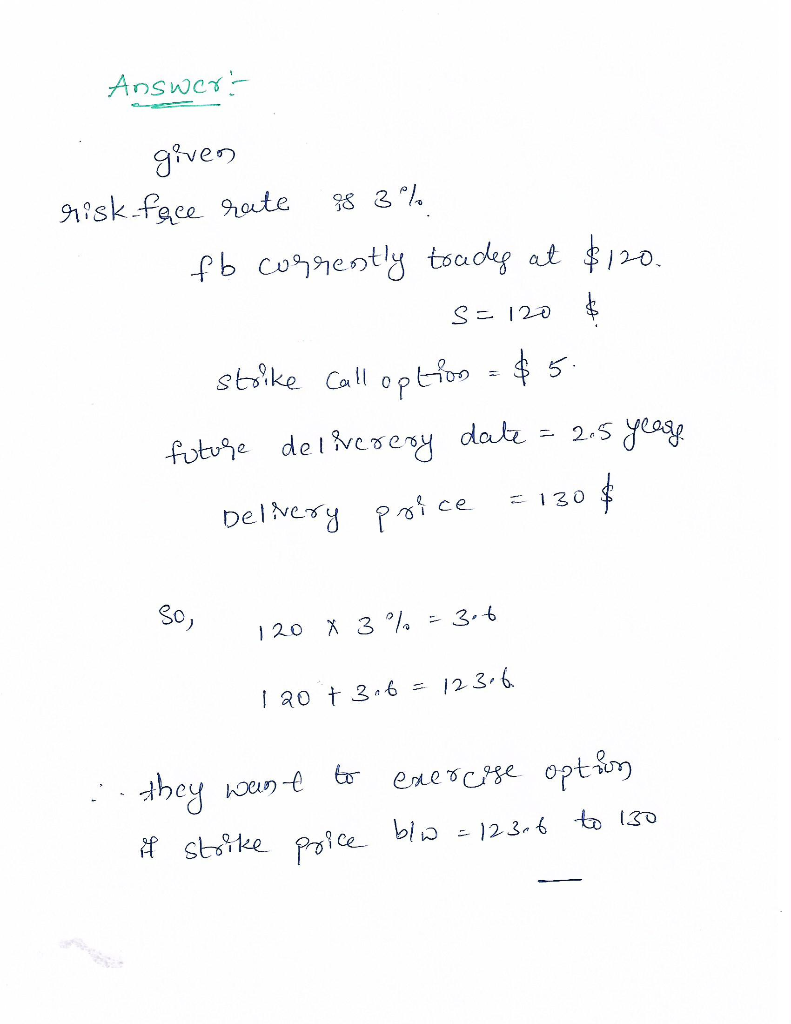##### Add Answer to: Assume the risk-free rate is 3% and will remain so for all time. Facebook (FB) currently...
Similar Homework Help Questions
• ### A futures price is currently \$25, its volatility (SD) is 30% per annum, and the risk-free...

A futures price is currently \$25, its volatility (SD) is 30% per annum, and the risk-free interest rate is 10% per annum. What is the value of a nine-month European call on the futures with a strike price of \$26 according to the BSM option pricing model?

• ### (11 Suppose that Facebook stock is currently priced at \$170 per share and you have bought...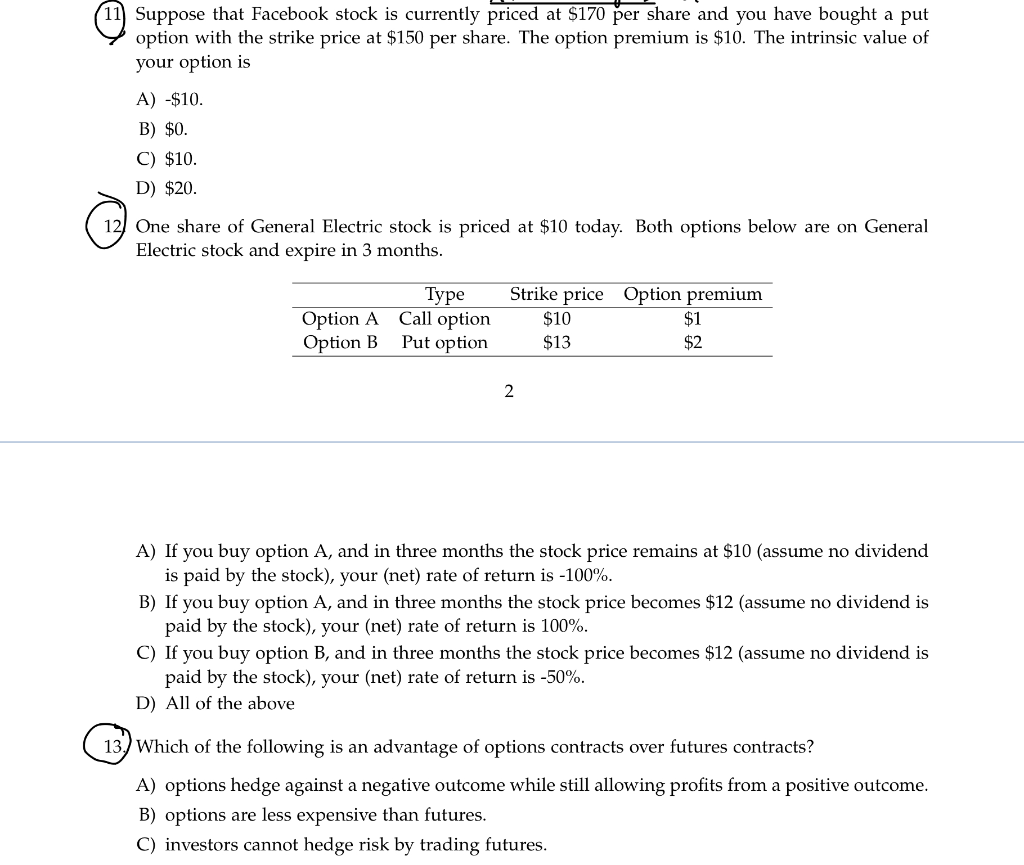(11 Suppose that Facebook stock is currently priced at \$170 per share and you have bought a put option with the strike price at \$150 per share. The option premium is \$10. The intrinsic value of your option is A) -\$10. B) \$0. C) \$10. D) \$20. One share of General Electric stock is priced at \$10 today. Both options below are on General Electric stock and expire in 3 months. Option A Option B Type Call option Put option...

• ### Problem1 A stock is currently trading at S \$40, during next 6 months stock price will increase to \$44 or decrease to \$32-6-month risk-free rate is rf-2%. a. [4pts) What positions in stock and T-...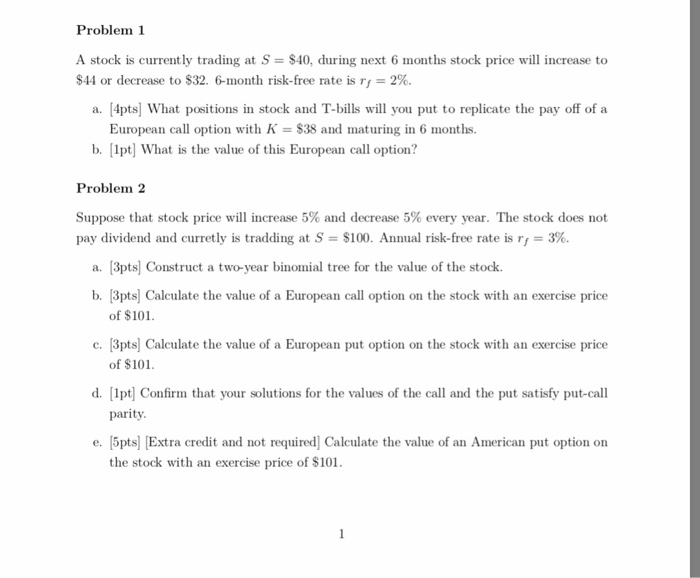Problem1 A stock is currently trading at S \$40, during next 6 months stock price will increase to \$44 or decrease to \$32-6-month risk-free rate is rf-2%. a. [4pts) What positions in stock and T-bills will you put to replicate the pay off of a European call option with K = \$38 and maturing in 6 months. b. 1pt What is the value of this European call option? Problem 2 Suppose that stock price will increase 5% and decrease 5%...

• ### Spot price 50 Strike price 50 Effective annual risk-free rate 1% Continuous Dividend Yield 0 Time...

Spot price 50 Strike price 50 Effective annual risk-free rate 1% Continuous Dividend Yield 0 Time to maturity 1 year European Call Option Premium 5.2 u 1.2 d 0.8 (a) Suppose you observe a call price of \$5.50. How can you arbitrage? A. Long call, long stock and borrow money. B. Short call, long stock and borrow money. C. Long call, short stock and lend money. D. Short call, short stock and lend money. (b) What is the present value...

• ### 1. (Put-call parity) A stock currently costs So per share. In each time period, the value...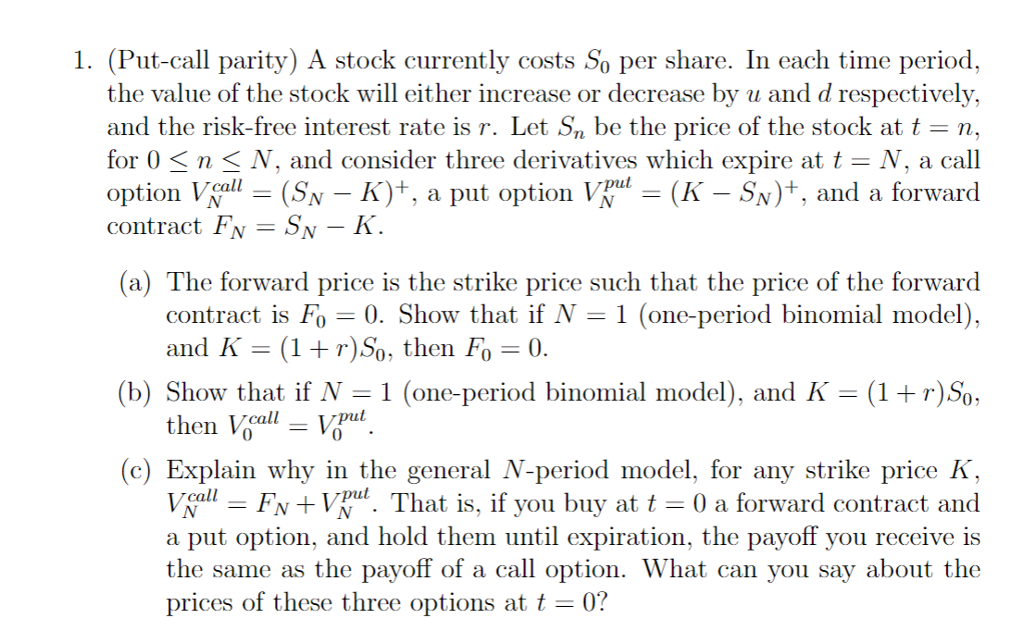1. (Put-call parity) A stock currently costs So per share. In each time period, the value of the stock will either increase or decrease by u and d respectively, and the risk-free interest rate is r. Let Sn be the price of the stock at t n, for O < n < V, and consider three derivatives which expire at t- N, a call option Vall-(SN-K)+, a put option Vpul-(K-Sy)+, ad a forward contract Fv -SN -K (a) The forward...

• ### Question 1 a. A stock price is currently \$30. It is known that at the end...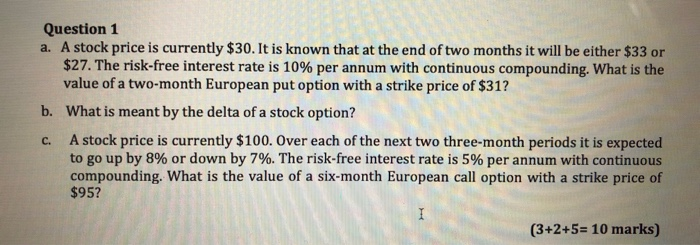Question 1 a. A stock price is currently \$30. It is known that at the end of two months it will be either \$33 or \$27. The risk-free interest rate is 10% per annum with continuous compounding. What is the value of a two-month European put option with a strike price of \$31? b. What is meant by the delta of a stock option? A stock price is currently \$100. Over each of the next two three-month periods it is...

• ### 1. (Put-call parity) A stock currently costs So per share. In each time period, the value...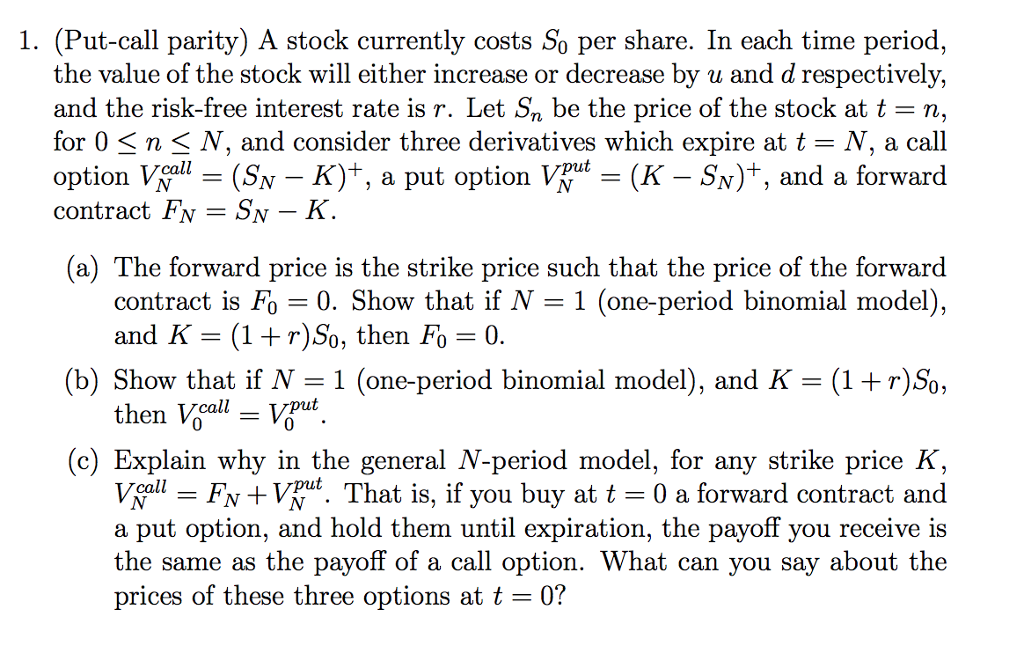1. (Put-call parity) A stock currently costs So per share. In each time period, the value of the stock will either increase or decrease by u and d respectively, and the risk-free interest rate is r. Let Sn be the price of the stock at t-n, for O < n < N, and consider three derivatives which expire at t - V, a cal option Voll-(SN-K)+, a put option VNut-(X-Sy)+, and a forward option VN(SN contract FN SN N) ,...

• ### Spot price 50 Strike price 50 Effective annual risk-free rate 1% Continuous Div...

Spot price 50 Strike price 50 Effective annual risk-free rate 1% Continuous Dividend Yield 0 Time to maturity 1 year European Call Option Premium 5.2 u 1.2 d 0.8 (a) Suppose you observe a call price of \$5.50. How can you arbitrage? A. Long call, long stock and borrow money. B. Short call, long stock and borrow money. C. Long call, short stock and lend money. D. Short call, short stock and lend money. (b) What is the present value...

• ### A) The spot price of the British pound is currently \$2.00. If the risk-free interest rate...

A) The spot price of the British pound is currently \$2.00. If the risk-free interest rate on 1-year Government bonds is 4% in the United States and 6% in the United Kingdom, what must be the forward price of the pound for delivery 1 year from now? B) Assume that the spot price of gold is \$1,500 per troy ounce, the risk-free interest rate is 2%, and storage and insurance costs are zero. 1) What should be the forward price...

• ### . The spot price per share is \$115 and the risk free rate is 5% per annum on a continuously compounded basis. The annual...

. The spot price per share is \$115 and the risk free rate is 5% per annum on a continuously compounded basis. The annual volatility is 20% and the stock does not pay any dividend. All options have a one-year maturity. In answering the questions below use a binomial tree with three steps. Each step should be one-third of a year. Show your work. 1.Using the binomial tree, compute the price at time 0 of a one-year European call option...

Free Homework App